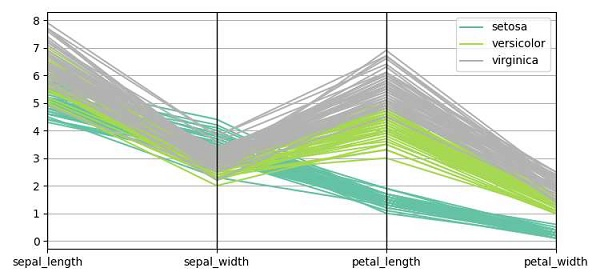# Plot parallel coordinates in Matplotlib

To plot parallel coordinates, we can take the following Steps −

• Load dataset iris using Seaborn (Need internet).

• Pass the loaded data into the parallel_coordinates() method, which will help in parallel plotting.

• To display the figure, use the show() method.

## Example

import matplotlib.pyplot as plt
from pandas.plotting import parallel_coordinates
import seaborn as sns
plt.rcParams["figure.figsize"] = [7.50, 3.50]
plt.rcParams["figure.autolayout"] = True
plt.show()